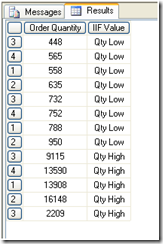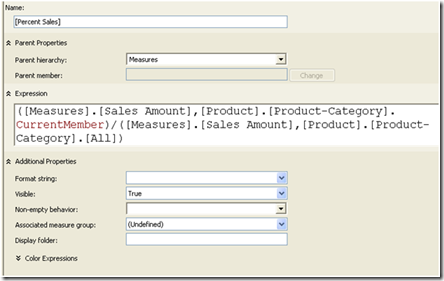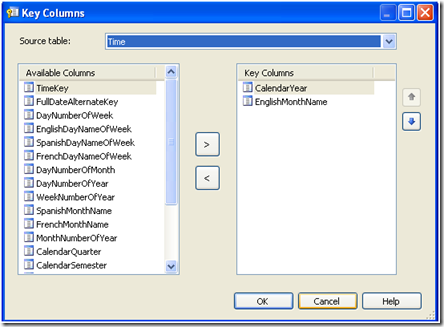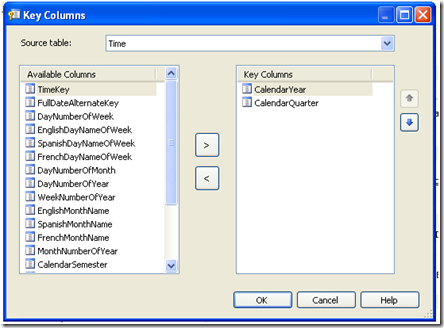### Exploring Additional Multi Dimensional Expression (MDX) Functions in SQL Server Analysis Services – Part 3

I recently wrote two articles on MDX in SQL Server Analysis Services

Exploring MDX (Multi Dimensional Expression) in SQL Server Analysis Services

Exploring Additional Multi Dimensional Expression (MDX) Functions in SQL Server Analysis Services – Part 2

This is the third and final part of the series and we will explore some additional functions of MDX:

1. IIF functions
returns one of the possible two values in a logical test. It can return either a string or numeric value.

`WITH MEMBER MEASURES.[IIF Value] ASIIF([Measures].[Order Quantity]>1000, "Qty High", "Qty Low")SELECT {[Measures].[Order Quantity],MEASURES.[IIF Value]} ON 0,[Order Date].[Calendar].[Calendar Quarter].MEMBERS ON 1FROM DemoCube`

In this query, we are displaying whether the quantity is low or high, according to the value for Order Quantity.We can change the condition if required.

2. Let us use IIF with string data

`WITH MEMBER [IIF String] ASIIF([Product].[Product-Category]=Bikes, "Common", "not common")SELECT {[Measures].[Sales Amount],[IIF String]} ON 0,[Product].[Product-Category].[Product Category Key].MEMBERS on 1FROM DemoCube`

3. Let us add a calculated member. The following query creates percent by using MDX and displays results:

`WITH MEMBER Measures.[Percent] AS([Measures].[Sales Amount],[Product].[Product-Category].CurrentMember)/([Measures].[Sales Amount],[Product].[Product-Category].[All]),FORMAT_STRING="Percent"SELECT Measures.[Percent] on 0,NON EMPTY [Product].[Product-Category].[Product Category Key].members on 1from democube`

This calculation can be kept for further usage if it is added from BIDS (Business Intelligence Development Studio) as followsHere we can give the format string to percent, if required. Later it can directly be used in MDX as follows:

`SELECT [Measures].[Percent Sales] ON0,NON EMPTY [Product].[Product-Category].[Product Category Key].members on1fromdemocube`

This will give the same result as the previous query, with the difference that we already have a calculated field.

4. Let us discuss different ways of using the COUNT function. The following query returns the number of hierarchies in a cube:

`WITH MEMBER MEASURES.[Count] ASdimensions.countSELECT Measures.[Count] ON 0FROM DemoCube`

5. The following query returns number of levels in the product categories:

`WITH MEMBER measures.[Count] AS[Product].[Product-Category].Levels.CountSelect Measures.[Count] ON 0FROM DemoCube`

6. The following query counts the number of cells in a set of members that consists of children of a category:

`WITH MEMBER measures.X AS[Product].[Product Category Key].children.countSELECT Measures.X ON 0FROM DemoCube`

We can exclude non empty cells in the following manner:

`WITH MEMBER measures.[Count] ASCount([Product].[Product Category Key].children, EXCLUDEEMPTY)SELECT Measures.[Count] ON 0FROM DemoCube`

7. Let us look at parallel period now.

In order to display the data in a more meaningful manner, I have added the following calculated fields in DSV (Data Source View):

Calendar Quarter Description: 'Q' + CONVERT(CHAR (1), CalendarQuarter) +' '+ 'CY ' +
CONVERT(CHAR (4), CalendarYear)

Calendar year description: 'CY '+ CONVERT(CHAR (4), CalendarYear)
and MonthName: EnglishMonthName+' '+ CONVERT(CHAR (4), CalendarYear)

I changed the time dimension to incorporate these changes as follows:

Attribute English Month Name

Key columns collection is as shown below:with Name column pointing to newly created MonthName

Similarly Calendar Quarter key columns is as followswith the Name column pointing to a Calendar Quarter description.

Calendar year’s name column points to Calendar Year Description

A parallel period returns a member from prior period in the same relative position as a specified member.

Two more properties need to be changed for English month name as follows:

OrderBy: AttributeKey
OrderByAttribute: Month Number of year

If we forget these settings, the order will be on character month and which will be misleading/

The following query returns parallel period for the month of July 2002 with a lag of 2 periods based on quarter level which is January 2002

`SELECT ParallelPeriod ([Order Date].[Calendar].[Calendar Quarter], 2, [Order Date].[Calendar].[English Month Name].&&[July])ON 0FROM DemoCube`

A similarly query

`SELECT ParallelPeriod ([Order Date].[Calendar].[Calendar Year], 1, [Order Date].[Calendar].[Calendar Quarter].&&)ON 0FROM DemoCube`

gives us result as Quarter 4 and year 2001. If we change the lag to 2 periods, we may not get the result in this case, as the starting year for the data is 2001

8. Parallel period is similar to Cousin function so let us discuss Cousin function.

This function returns child member with same relative position under a parent member as the given child member.

The following query displays cousin of 3rd quarter of year 2001 based on year level in 2002, which gives quarter 1 in 2003

`SELECT Cousin( [Order Date].[Calendar].[Calendar Quarter].&&,[Order Date].[Calendar].[Calendar Year].&) ON 0FROM DemoCube`

In these 3 articles, we discussed what is MDX, how queries can be used to view data from multi dimensional cubes and different clauses for MDX queries. We also discussed some functions available with MDX (sum, avg, prevmember, count,min, max, topcount) and how formatting can be provided, so that the result looks user friendly. We discussed how calculated members can be created and displayed. We also discussed some more functions like IIF, Parallel Period and Cousin.